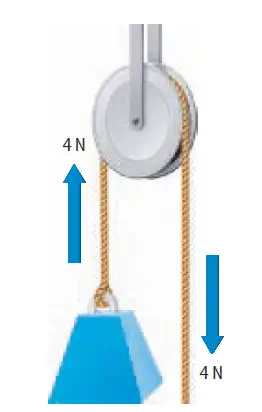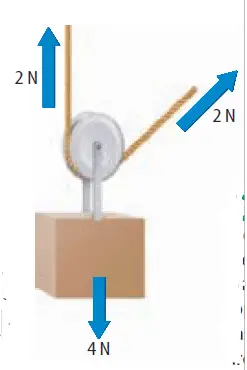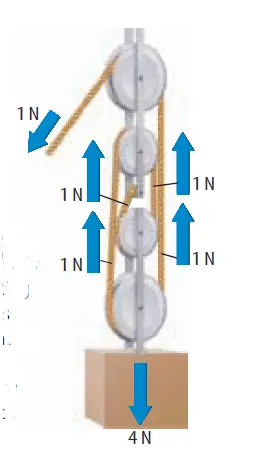# Types of pulleys and how they differ in their operation

In this post, we will discuss three types of pulleys, and how they operate, and how they differ in their operation and utilities. The types we will discuss are (1) Fixed Pulleys, (2) Movable Pulleys, (3) the Block and Tackle.

## Fixed Pulleys

A fixed pulley, such as the one in Figure 1, is attached to something that doesn’t move, such as a ceiling or wall. A fixed pulley changes only the direction of force, it doesn’t multiply the input force. Hence, the IMA of a fixed pulley is 1.figure 1: [A fixed pulley changes only the direction of a force. You need to apply an input force of 4 N to lift the 4-N weight.]

### Example of a fixed pulley

Elevator pulley is a fixed pulley – The cable attached to an elevator passes over a fixed pulley at the top of the elevator shaft.

## Movable Pulleys

A pulley in which one end of the rope is fixed and the wheel is free to move is called a movable pulley.

Unlike a fixed pulley, a movable pulley does multiply force.

Suppose a 4-N weight is hung from the movable pulley in Figure 2. The ceiling acts like someone helping you to lift the weight. The rope attached to the ceiling will support half of the weight (2 N here). You need to exert only the other half of the weight (2 N here) – to support and lift the weight.figure 2: With a movable pulley, the attached side of the rope supports half of the load. Hence you need to apply a force that is half of the weight only.

The output force exerted on the weight is 4 N, and the applied input force is 2 N. Therefore the IMA of the movable pulley is 2.

For a fixed pulley, the distance you pull the rope downward equals the distance the weight moves upward. But, for a movable pulley, the distance you pull the rope upward is twice the distance the weight moves upward.

## The Block and Tackle

A system of pulleys consisting of fixed and movable pulleys is called a block and tackle.

Figure 3 shows a block and tackle made up of two fixed pulleys and two movable pulleys.[figure 3: In a block-and-tackle system, the 4-N weight is divided equally among each supporting rope segment. In this case, four rope segments are supporting the weight. So you have to apply only a 1-N force to lift the 4 N weight.]

If a 4-N weight is suspended from the movable pulley of the block and tackle in figure 3, each rope segment supports one-fourth of the weight, reducing the input force to 1 N.

The IMA of a pulley system is equal to the number of rope segments that support the weight. [IMA means Ideal Mechanical Advantage]

The block and tackle shown in Figure 3 has an IMA of 4.

The IMA of a block and tackle can be increased by increasing the number of pulleys in the pulley system.

See also  How to use Pascal’s principle (or Pascal's law) in Hydraulic Car Lift?
Scroll to top
error: physicsTeacher.in